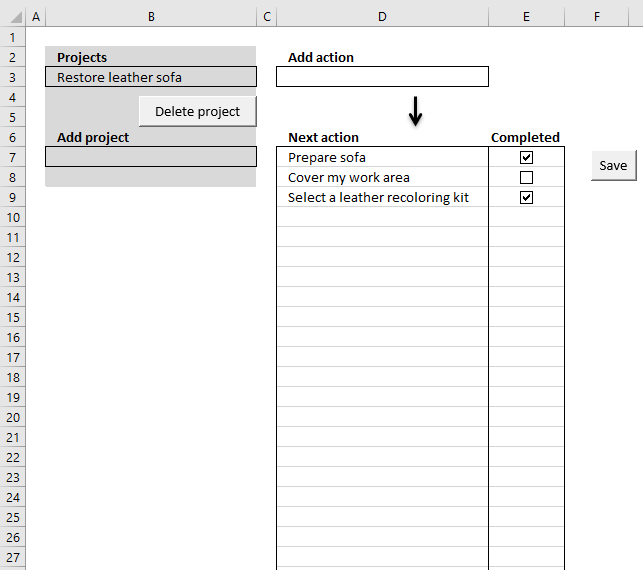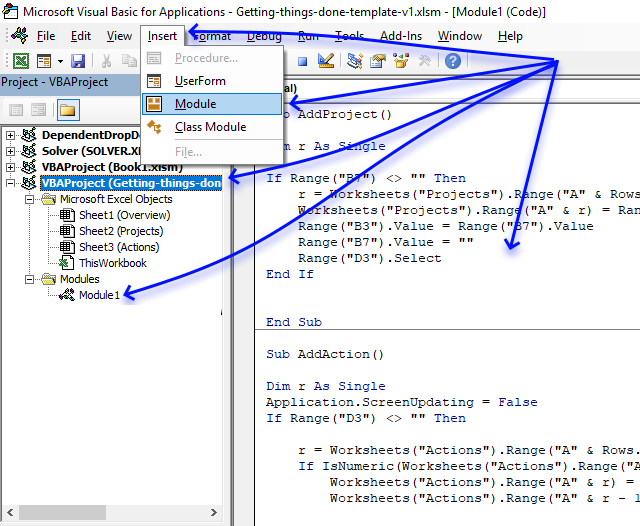Author: Oscar Cronquist Article last updated on August 21, 2019In this article, I am going to demonstrate a simple workbook where you can create or delete projects and add "next" actions to each project. You can also mark actions completed. The ideas here are based on the "Getting things done" mindset.

"The Getting Things Done method rests on the idea that a person needs to move tasks out of the mind by recording them externally, so the mind is free from the job of remembering the tasks that need to be completed. One can concentrate on performing the tasks, instead of remembering."
Source: Wikipedia

### Add a project

Simply enter a project name in cell B7 and then press Enter, the project now appears in the drop-down list in cell B3.

The following event code monitors if cell B3 is changed in worksheet "Overview" and runs macro AddProject.

```'Event code located in the "Overview" worksheet module and is triggered if cell is changed.
Private Sub Worksheet_Change(ByVal Target As Range)

'Check if changed cell is B3
If Target.Address = "\$B\$3" Then

'Start macro RefreshList
Call RefreshList

End If

'Check if changed cell is B7
If Target.Address = "\$B\$7" Then

End If

'Check if changed cell is D3
If Target.Address = "\$D\$3" Then

End If

End Sub

```

This macro populates the list on worksheet Projects with the value entered in cell B7.

```'Name macro

'Dimension variable and declare datatypes
Dim r As Single

'Check if cell B7 is not empty
If Range("B7") <> "" Then

'Calculate the cell row below the last value in column A in worksheet Projects
r = Worksheets("Projects").Range("A" & Rows.Count).End(xlUp).Row + 1

'Copy cell B7 and paste to first empty value
Worksheets("Projects").Range("A" & r) = Range("B7").Value

'Copy cell B7 and paste to cell B3
Range("B3").Value = Range("B7").Value

'Clear cell B7
Range("B7").Value = ""

'Select cell D3
Range("D3").Select
End If

End Sub

```

### Delete a project

Select a project in the drop down list in cell B3. Click "Delete project" button, a dialog box warns you that all the actions that belong to the project also will be removed.

Click Yes to remove the selected project.

```'Name macro
Sub DeleteProject()

'Dimension variables and declare data types
Dim rP As Single, rA As Single

'Save row number of last non empty cell in worksheet Projects to variable rP
rP = Worksheets("Projects").Range("A" & Rows.Count).End(xlUp).Row

'Save row number of last non empty cell in worksheet Projects to variable rA
rA = Worksheets("Actions").Range("A" & Rows.Count).End(xlUp).Row

'Show Messagebox and prompt for Yes or No, save answer to variable Ans
Ans = MsgBox("Delete project: " & Range("B3") & "? All actions are also deleted!", vbYesNo)

'Check if variable Ans is equal to 6
If Ans = 6 Then

'Check if variable rP is larger than 1
If rP > 1 Then

'Create a loop
Do

'Check which row are equal to value in cell B3
If Worksheets("Projects").Range("A" & rP) = Range("B3").Value Then

'Delete row which are equal to value in cell B3
Worksheets("Projects").Range("A" & rP).Delete Shift:=xlUp

'Stop loop
Exit Do
End If

'Subtract value in variable rP with 1
rP = rP - 1

'Continue loop until variable rP is equal to 1
Loop Until rP = 1

'Check if variable rA is larger than 1
If rA > 1 Then

'Create loop
Do

'Check i value in column B in worksheet Actions is equal to value in cell B3 (worksheet Overview´)
If Worksheets("Actions").Range("B" & rA) = Range("B3").Value Then

'Delete row
Worksheets("Actions").Range(rA & ":" & rA).Delete Shift:=xlUp
End If

'Subtract value in variable rA with 1
rA = rA - 1

'Continue loop until variable rA is equal to 1
Loop Until rA = 1
End If
End If

'Clear value in cell B3
Range("B3").Value = ""
End If
End Sub

```

### Select a project

The drop-down list in cell B3 allows you to select a project and see the corresponding actions in column D, the following macro makes that happen.

There is also event code that runs this macro when the value in cell B3 is changed, that code is shown above under header "Add a project".

The macro lists corresponding actions to selected project and checkboxes next to actions that allows you to mark actions completed.

```'Name macro
Sub RefreshList()

'Dimension variables and declare data types
Dim rA As Single, rO As Single
Dim Rng As Range
Dim CLeft As Double, CTop As Double, CHeight As Double, CWidth As Double

'Disable window to refresh
Application.ScreenUpdating = False

'Save Selection to object Rng
Set Rng = Selection

Range("D7:D" & Rows.Count) = ""

'Save last nonempty cell's row number to variable rA
rA = Worksheets("Actions").Range("B" & Rows.Count).End(xlUp).Row

'Delete all checkboxes on active worksheet
ActiveSheet.CheckBoxes.Delete

'Check if cell B3 (sheet Overview) is not empty and variable rA is above 1
If Range("B3").Value <> "" And rA > 1 Then

'Create a loop
Do

'Check if project in sheet Actions is equal to chosen project in cell B3 (sheet Overview) based on row number in variable rA
If Worksheets("Actions").Range("B" & rA) = Range("B3").Value Then

'Save row number of first empty cell to variable rO
rO = Worksheets("Overview").Range("D" & Rows.Count).End(xlUp).Row + 1

'Use row number in variable rO and rA to copy corresponding action to Overview sheet
Worksheets("Overview").Range("D" & rO) = _
Worksheets("Actions").Range("C" & rA).Value

'Save number of points to left border from cell in column E based on row number in variable rO, to variable CLeft
CLeft = Cells(rO, "E").Left

'Save number of points to top border from cell in column E based on row number in variable rO, to variable CTop
CTop = Cells(rO, "E").Top

'Save cell height in points of cell in column E based on row number in variable rO, to variable CTop
CHeight = Cells(rO, "E").Height

'Save height in points of cell in column E based on row number in variable rO, to variable CTop
CWidth = Cells(rO, "E").Width

'Add checkbox based on points saved in variables CLeft, CWidth, CTop, CHeight, this also selects the cell
ActiveSheet.CheckBoxes.Add(CLeft + CWidth / 2 - 8, CTop, CWidth, CHeight).Select

'With - With End statement allows you to write shorter code by referring to an object only once instead of using it with each property.
With Selection

'Clear caption
.Caption = ""

'Check if checkbox is checked or unchecked in list
If Worksheets("Actions").Range("D" & rA).Value = 1 Then
.Value = 1
Else
.Value = xlOff
End If
End With
End If

'Subtract variable rA with 1
rA = rA - 1

'Continue loop until rA is equal to 1
Loop Until rA = 1
End If

'Select Rng object
Rng.Select

'Show changes to user
Application.ScreenUpdating = True
End Sub
```

### Add actions to a project

Enter the name of the action you want to add in cell D3. Event code makes sure that macro AddAction is executed when a new value is enter in cell D3.

```'Name macro

'Dimension variables and data types
Dim r As Single

'Disable screen updating while macro is executed, this speeds up the macro
Application.ScreenUpdating = False

'Check if cell D3 is not empty
If Range("D3") <> "" Then

'Save row number of first empty cell to variable r
r = Worksheets("Actions").Range("A" & Rows.Count).End(xlUp).Row + 1

'Check if cell above first empty cell contains a numerical value
If IsNumeric(Worksheets("Actions").Range("A" & r - 1)) Then

'Save number + 1 to first empty cell in worksheet actions
Worksheets("Actions").Range("A" & r) = _
Worksheets("Actions").Range("A" & r - 1) + 1

Else
'Save 1 to first empty cell
Worksheets("Actions").Range("A" & r) = 1

End If

'Copy value in cell B3 to cell in column B in worksheet actions based on value in variable r
Worksheets("Actions").Range("B" & r) = Range("B3").Value

'Copy value in cell D3 to cell in column C in worksheet actions based on value in variable r
Worksheets("Actions").Range("C" & r) = Range("D3").Value

'Clear cell D3
Range("D3").Value = ""

End If

'Select cell D3
Range("D3").Select

'Show changes to user
Application.ScreenUpdating = True

'Run macro RefreshList
Call RefreshList

End Sub
```

### Mark an action completed

The Save button allows you to save completed actions, the button is linked to macro ActionComp.

```'Name macro
Sub ActionComp()

'Dimension variables and declare data types
Dim rO As Single, rA As Single

'Save cell D7 (sheet Overview) to object checkO
Set checkO = Worksheets("Overview").Range("D7")

'Save cell C1 (sheet Actions) to object checkA
Set checkA = Worksheets("Actions").Range("C1")

'Save row number of first empty cell in column C (sheet Actions) to variable rA
rA = Worksheets("Actions").Range("C" & Rows.Count).End(xlUp).Row

'Save row number of first empty cell in column D (sheet Overview) to variable rO
rO = Worksheets("Overview").Range("D" & Rows.Count).End(xlUp).Row

'Iterate through 0 (zero) to value in variable rO minus 7
For o = 0 To rO - 7

'Iterate through 1  to value in variable rA minus 1
For a = 1 To rA - 1

'Check if value in column D (sheet Overview) based on row number in variable o is equal to value in column C (sheet Actions) based on row number in variable a AND value in cell B3 is equal to value in column B (sheet Actions) based on row number in variable a
If checkO.Offset(o, 0).Value = checkA.Offset(a, 0).Value _
And Worksheets("Overview").Range("B3").Value = checkA.Offset(a, -1).Value Then

'Save checkbox value to column D (sheet Actions) based on row number in variable a
checkA.Offset(a, 1).Value = ActiveSheet.CheckBoxes(o + 1).Value
End If
Next a
Next o

End Sub
```

### Where to put the macro and event code?1. Press Alt+ F11 to open the Visual Basic Editor.
2. Double click the workbook you want to attach the code to in the Project Explorer.
3. Click on "Insert" on the menu.
4. Click on "Module" to insert a module to the selected workbook.
5. Paste code to code module.

The event code is not saved to a code module, it is saved to a sheet module named Sheet1 (Overview) object, simply double click on it to show the sheet module. Paste event code to the sheet module.

Note, save the workbook with the file extension *.xlsm (macro-enabled) workbook so the code stays attached to the workbook.